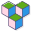CppBuzz# C program to find Voltage using Ohm's Law

This C program takes value of I & R from user as input and then calculate V i.e voltage using formula I * R.

``````#include <stdio.h>
#include <conio.h>

int main ()
{
printf("\n#### C Program to find Voltage using Ohm's Law\n");

float I, R, V;

printf("\n Enter the value of Current I = ");
scanf("%f",&I);
printf("\n Enter the value of Resistance R = ");
scanf("%f",&R);

V=I*R;

printf("\n The required Voltage = %f", V);

return 0;
}
```
```

## Finding the value of Voltage when I = 0.5 and R = 10